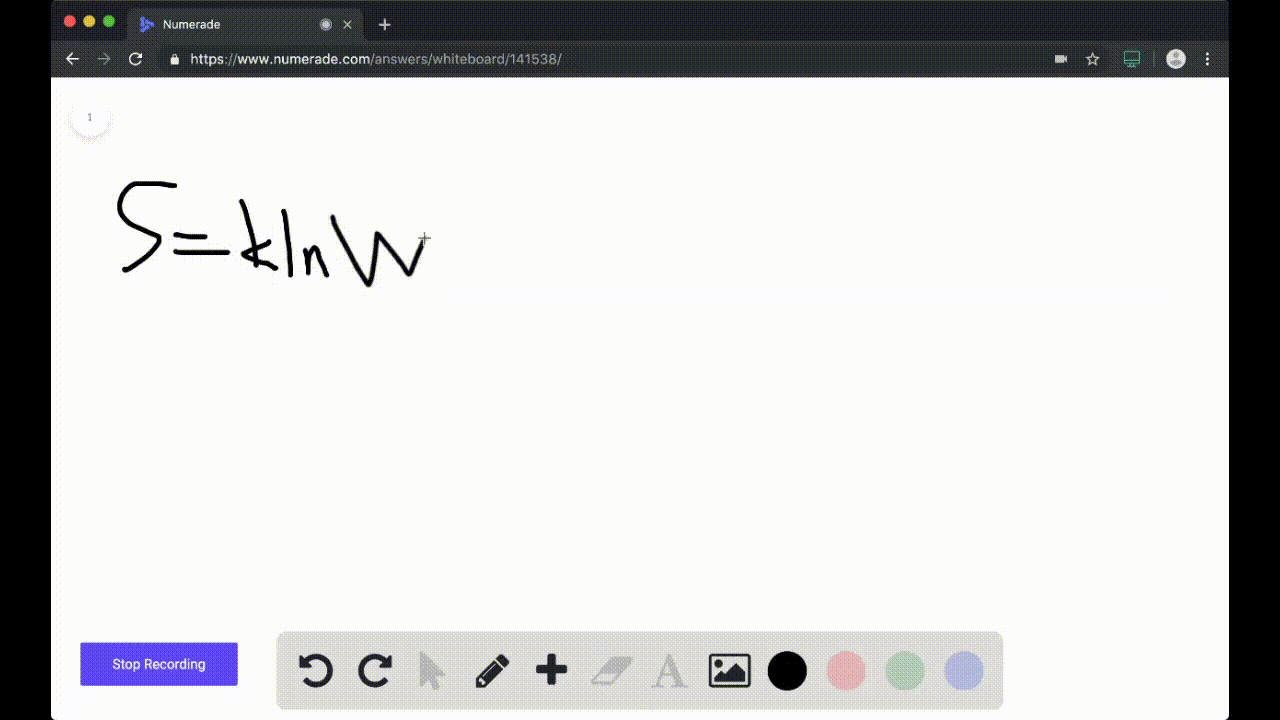### Calculate the entropy of each state and rank the …

04:04### Our team of expert educators are currently working on this.

#### Get notified when this problem is solved.

Our educator team will work on creating an answer for you in the next 6 hours.

Problem 103

Review the box in this chapter entitled Chemistry in Your Day: Making a Nonspontaneous Process Spontaneous. The hydrolysis of ATP, shown in Problem $91,$ is often used to drive nonspontaneous processes - such as muscle contraction and protein synthesis-in living organisms. The nonspontaneous process to be driven must be coupled to the ATP hydrolysis reaction. For example, suppose the nonspontaneous process is $\mathrm{A}+\mathrm{B} \longrightarrow \mathrm{AB}$ $\left(\Delta G^{\circ}\right.$ positive). The coupling of a nonspontaneous reaction such as this one to the hydrolysis of ATP is often accomplished by the mechanism:
$$\mathrm{A}+\mathrm{ATP}+\mathrm{H}_{2} \mathrm{O} \longrightarrow \mathrm{A}-\mathrm{P}_{1}+\mathrm{ADP}$$
$$\frac{\mathrm{A}-\mathrm{P}_{1}+\mathrm{B} \longrightarrow \mathrm{AB}+\mathrm{P}_{1}}{\mathrm{A}+\mathrm{B}+\mathrm{ATP}+\mathrm{H}_{2} \mathrm{O} \longrightarrow \mathrm{AB}+\mathrm{ADP}+\mathrm{P}_{1}}$$
As long as $\Delta G_{\mathrm{rxn}}$ for the nonspontaneous reaction is less than $30.5 \mathrm{kJ},$ the reaction can be made spontaneous by coupling in this way to the hydrolysis of ATP. Suppose that ATP is to drive the reaction between glutamate and ammonia to form glutamine:
\begin{equation}\begin{array}{l}{\text { a. Calculate } K \text { for the reaction between glutamate and ammo- }} \\ {\text { nia. (The standard free energy change for the reaction is }} \\ {+14.2 \mathrm{kJ} / \mathrm{mol} . \text { Assume a temperature of } 298 \mathrm{K} . \text { . }} \\ {\text { b. Write a set of reactions such as those given showing how the }} \\ {\text { glutamate and ammonia reaction can couple with the hy- }} \\ {\text { drolysis of ATP. What is } \Delta G_{\mathrm{rn}}^{\circ} \text { and } K \text { for the coupled reaction? }}\end{array}\end{equation}

A. $3.24 \times 10^{-3}$
B. $7.20 \times 10^{2}$

## Discussion

You must be signed in to discuss.

## Video Transcript

No transcript available